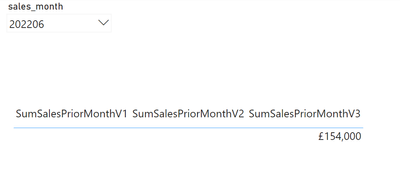cancel
Showing results for
Did you mean:Anonymous
Not applicable

## Unexpected results when using FILTER as a filter argument within CALCULATE

Hi,

I’m hoping for some help in understanding some unexpected results when using FILTER as a filter argument.

A high-level summary is that I was hoping to create a measure which returns the total sales from the prior month, on a page with a single select enabled slicer on month. For example, if the slicer is set to 202208 then measure would return total sales for 202207, if slicer is set to 202212 then measure returns total sales for 202211 (please note this month column is an integer where the value is YYYYMM).

There exist two tables, Sales and DatesTable, with a 1:M relationship on the sales_month column. Furthermore, the DatesTable is DAX calculated from the distinct month entries of Sales, and has a calculated column for descending rank.I first created a measure to return the distinct desc_rank for the selected month:

``SelectedMonthRank = SELECTEDVALUE(DatesTable[desc_rank])``

As measures cannot directly be used in boolean expression filter arguments I assumed I would need to use a nested FILTER in CALCULATE (V1) but this did not return the expected value, and instead returned blank. Likewise, initially moving the measure to a variable (V2) still returned blank. And it was only when removing the FILTER filter argument entirely (V3) that the expected results was produced.

``````SumSalesPriorMonthV1 =
CALCULATE(SUM(Sales[sales_count]),
REMOVEFILTERS(DatesTable),
FILTER(DatesTable, DatesTable[desc_rank] = [SelectedMonthRank] + 1))

SumSalesPriorMonthV2 =
var SelectedRank = [SelectedMonthRank]
RETURN
CALCULATE(SUM(Sales[sales_count]),
REMOVEFILTERS(DatesTable),
FILTER(DatesTable, DatesTable[desc_rank] = SelectedRank + 1))

SumSalesPriorMonthV3 =
var SelectedRank = [SelectedMonthRank]
RETURN
CALCULATE(SUM(Sales[sales_count]),
REMOVEFILTERS(DatesTable),
DatesTable[desc_rank] = SelectedRank + 1)
``````I therefore assume either my syntax or model is incorrected and wanted to understand which changes should be made so that I can use FILTER as a filter argument correctly if required for more complex filters (such as filtering with an OR criteria which I also do not think is achievable through the boolean expression filter arguments).

Many thanks 🙂

1 ACCEPTED SOLUTIONSuper User

The issue is because the REMOVEFILTERS is not being applied to the FILTER statement, it is being applied to the SUM. When you are manipulating the filter context, both FILTER and REMOVEFILTERS are being applied to the Dates table at the same time, so when FILTER is checking the Dates table the slicer is still in effect and it will return no rows, hence you get a blank result.

It is not usually necessary to include FILTER as a filter condition to CALCULATE, you can add the filters much more conveniently to the CALCULATE statement itself. You can add multiple conditions, against multiple tables, and you can combine conditions with and ( && ) and or ( || ) e.g.

``````Some measure =
CALCULATE (
SUM ( 'Table1'[Sales'] ),
'Table1'[Col1] = "X"
|| 'Table1'[Col2] = 3,
'Table2'[Col1] = "Y"
&& 'Table2'[Col2] = 4
)
``````

Also, I would recommend that you create a proper date table and either convert your current column to a date as the first of the month, or create a new column with the date of the first of the month. Not only is this best practice but it will allow you to take advantage of all the time intelligence functions like PREVIOUSMONTH, which would be handy in this case.Super User

The issue is because the REMOVEFILTERS is not being applied to the FILTER statement, it is being applied to the SUM. When you are manipulating the filter context, both FILTER and REMOVEFILTERS are being applied to the Dates table at the same time, so when FILTER is checking the Dates table the slicer is still in effect and it will return no rows, hence you get a blank result.

It is not usually necessary to include FILTER as a filter condition to CALCULATE, you can add the filters much more conveniently to the CALCULATE statement itself. You can add multiple conditions, against multiple tables, and you can combine conditions with and ( && ) and or ( || ) e.g.

``````Some measure =
CALCULATE (
SUM ( 'Table1'[Sales'] ),
'Table1'[Col1] = "X"
|| 'Table1'[Col2] = 3,
'Table2'[Col1] = "Y"
&& 'Table2'[Col2] = 4
)
``````

Also, I would recommend that you create a proper date table and either convert your current column to a date as the first of the month, or create a new column with the date of the first of the month. Not only is this best practice but it will allow you to take advantage of all the time intelligence functions like PREVIOUSMONTH, which would be handy in this case.